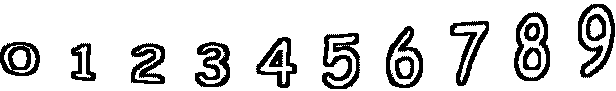1 + 10 =        3 + 7 =1 + 8 =        1 + 0 =        0 + 5 =        7 + 1 = 10 + 2 =        2 + 0 =        7 + 4 =        1 + 4 =